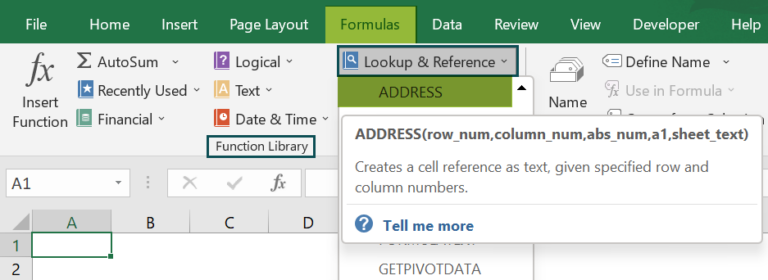Article byExcelMojo Team## What Is ADDRESS Function In Excel?

The ADDRESS Function in Excel provides the cell address or cell reference of a selected cell w.r.t the specified row and column. We can combine it with other functions to directly refer to a cell. The function will return the cell address as a text string.

The ADDRESS Function in Excel is an inbuilt “Lookup and Reference” function, so we can enter the formula directly in the worksheet or insert it from the “Function Library”.

For example, we will apply the ADDRESS Function in Excel to get the cell’s address.

Select cell A1, enter the formula =ADDRESS(1,1), and press the “Enter” key.

The output is “\$A\$1”, as shown above, i.e., the address is 1st Row in 1st Column.

###### Key Takeaways
• The ADDRESS Function in Excel helps users find the cell address in a dataset by giving the row and column numbers as inputs.
• We get the “#VALUE!” error,
• If the row or column number argument is less than 1.
• If the function arguments are non-numeric values.
• Once the ADDRESS formula is set, if the cell value with the formula changes or is copied or moved to other cells, the function will update the formula automatically.
• We can use the ADDRESS Function along with the ROW(), COLUMN(), and INDIRECT() functions.

The Syntax of the ADDRESS Excel formula is,

The arguments of the ADDRESS Excel formula are,

• row_num: It is the mandatory argument. It is a number/value that represents the row number.
• column_num: It is the mandatory argument. It is a number/value that represents the column number.
• abs_num: It is an optional argument. It is a number/value that represents the type of reference to return, whether absolute, relative, or mixed, as follows:
• a1: It is the optional argument. It is a logical value where both the columns and rows are labeled numerically, or the cell address is given. It can either be TRUE [A1 style] or FALSE [R1C1 style]. By default, it will return TRUE.
• sheet_text: It is the optional argument. It is the worksheet name of the target cell. By default, it will take the current worksheet.

### How to Use ADDRESS Excel Function?

We can use the ADDRESS function in Excel in 2 methods, namely,

1. Access from the Excel ribbon.
2. Enter in the worksheet manually.

#### Method #1 – Access from the Excel ribbon

First, choose an empty cell for the output → select the “Formulas” tab → go to the “Function Library” group → click the “Lookup & Reference” drop-down → select the “ADDRESS” function as shown below.

The “Function Arguments” windowappears. Enter the value in the row_num”, “column_num”, “abs_num”, “a1”, andsheet_textfields, accordingly, and click “OK”, as shown below.

#### Method #2 – Enter in the worksheet manually

1. Select an empty cell for the output.
2. Type =ADDRESS( in the selected cell. [Alternatively, type =A and double-click the ADDRESS function from the list of suggestions shown by Excel.]
3. Enter the arguments accordingly and close the brackets.
4. Press the “Enter” key.

We will calculate the cell addresses using a basic example.

In the table, the data is,

• Column A contains the Row_num.
• Column B contains the Column_num.
• Column C contains the abs_num.
• Column D contains the a1.
• Column E contains the Sheet_text.
• Column F displays the Output.

The steps to get the cell address using the ADDRESS Function in Excel example are as follows:

1. Select cell F2, enter the formula =ADDRESS(A2 i.e., the row_num value, put a comma ‘,’.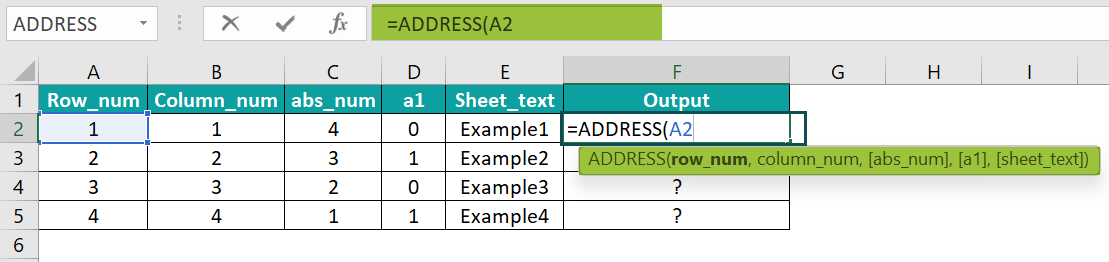2. Enter the column_num value i.e., B2, the formula now is =ADDRESS(A2,B2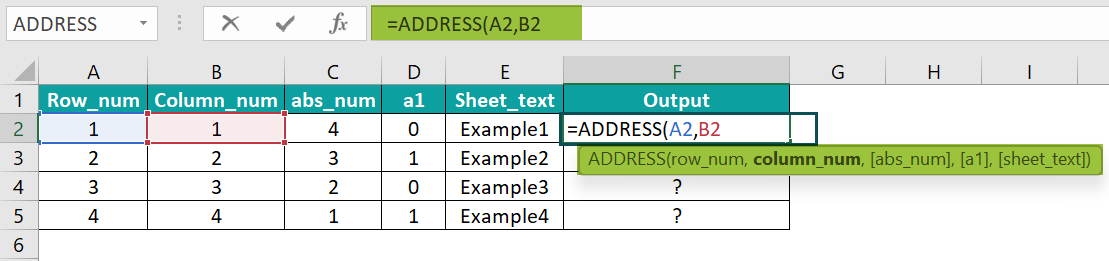3. Put a comma ‘,’, and enter the abs_num value, i.e., C2, the formula now is =ADDRESS(A2,B2,C2, and put a comma ‘,’.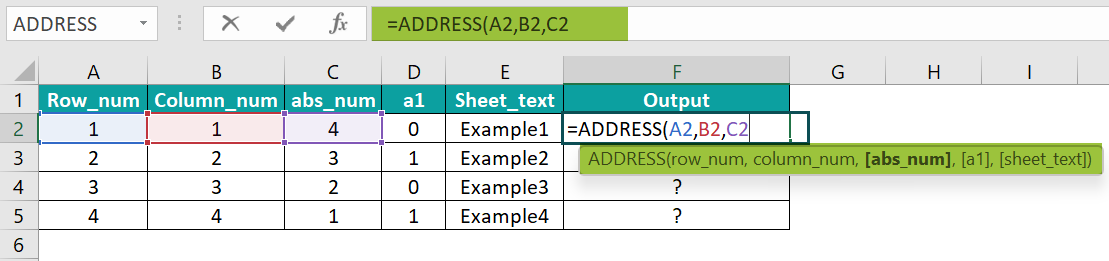4. Enter the a1 value i.e. D2, the formula now is =ADDRESS(A2,B2,C2,D2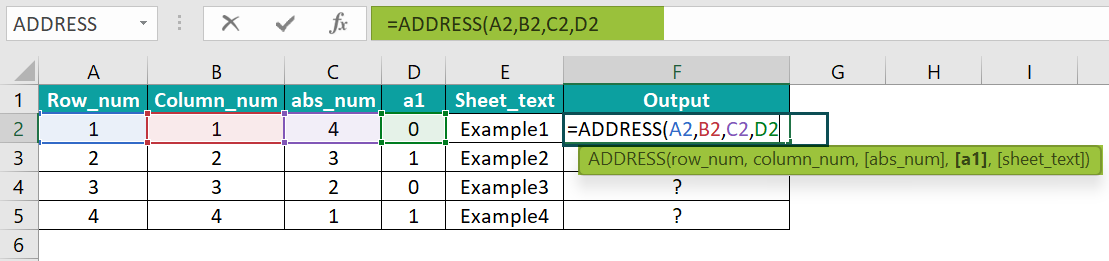5. Put a comma ‘,’, enter the sheet_text value i.e., E2, and close the brackets. The complete formula is =ADDRESS(A2,B2,C2,D2,E2)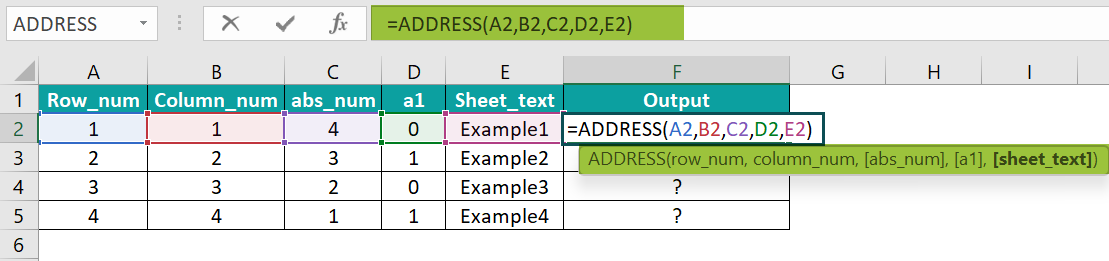6. Press the “Enter” key. The output is Example1!RC, as shown below.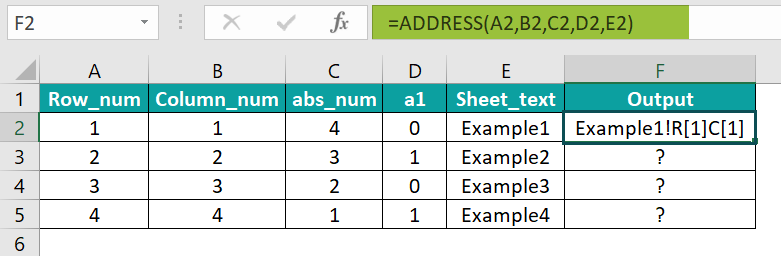7. Drag the formula from cell F2 to F5 using the fill handle.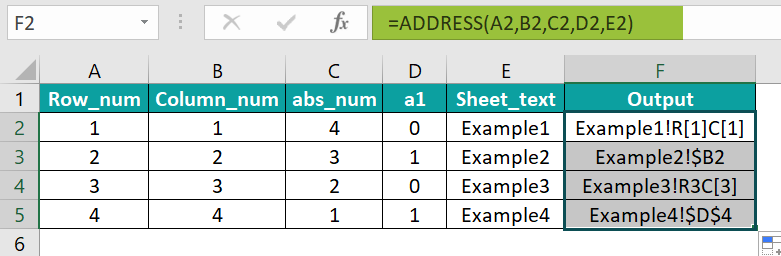The output is shown above in the absolute and relative styles.

### Examples

We will understand some advanced scenarios by using ADDRESS function in Excel.

#### Example #1

In the table, the data is,

• Column A contains the Row_num.
• Column B contains the Column_num.
• Column C displays the Output.

The steps to get the cell address by using the ADDRESS Function in Excel are as follows:

• 1: Select cell C2 and enter the formula =ADDRESS(A2,B2).
• 2: Press the “Enter” key. The result is “\$A\$4”, as shown below.
• 3: Drag the formula from cell C2 to C5 using the fill handle.

The output is shown above. Only the mandatory argument inputs return the default style output.

#### Example #2

We will get the cell addresses using the ADDRESS() and the INDIRECT function.

[Note: The INDIRECT function gets the value stored in the cell address. We get the cell reference from the ADDRESS function and the actual value through a reference from the INDIRECT function.]

In the table, the date is,

• Column A contains the Fruits.
• Column B contains the Price.
• Column E displays the Output.

The procedure to find the value using the INDIRECT() and the ADDRESS() functionsare as follows:

• First, select cell E2, enter the formula =INDIRECT(ADDRESS(4,2)), and press the “Enter” key.

The output is “\$200”, as shown above. The cell address is 4th row, 2nd column, and the value is “\$200” that the INDIRECT function returned.

#### Example #3

We will calculate the first and second cell address values using the ADDRESS Function in Excel along with the ROW() function and COLUMN() function.

In the table, the data,

• Column A contains the Items.
• Column B contains the Price.
• Column E displays the Output.

The steps to find the value by the ADDRESS Function in Excel are as follows:

• Step 1: Select cell E2, enter the formula =ADDRESS(ROW(B3:B5),COLUMN(B3:B5)), and press the “Enter” key. The result is “\$B\$3”, as shown below.
• Step 2: Select cell E3, enter the formula =ADDRESS(ROW(B3:B5)+ROWS(B3:B5)-1,COLUMN(B3:B5)+COLUMNS(B3:B5)-1), and press the “Enter” key. The output is “\$B\$5”, as shown below.

[Note: In the E3 cell’s formula, the row_num is ROW(B3:B5)+ROWS(B3:B5)-1 and the column_num as COLUMN(B3:B5)+COLUMNS(B3:B5)-1.]

### Important Things to Note

• The “abs_num” value is set according to the following parameters:
• The reference style can be True or False. Now, the default reference style of the function is “A1” if no value is entered for the argument.
• To change the cell reference style from the usual Excel style,
• Select FileOptions, to open the “Excel Options” window.
• On the left side of the “Excel Options” window, select “Formulas” from the list.
• On the right side, in the “Working with formulas” group, check/tick the “R1C1 reference style” checkbox, as shown below.

1. What does the ADDRESS function do in Excel?

The ADDRESS Function in Excel locates the cell address of the given row and column numbers.
For example, to find the address of the cells A1, A2, B1, and B2, the formulas are:
Select cell A1, enter the formula =ADDRESS(1,1), and the result is “\$A\$1”.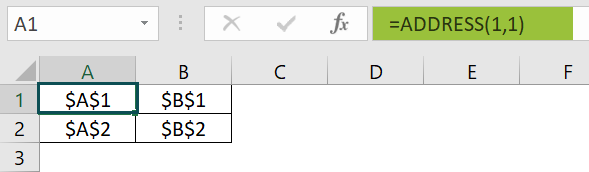In cell B1, enter the formula =ADDRESS(1,2), and the result is “\$B\$1”.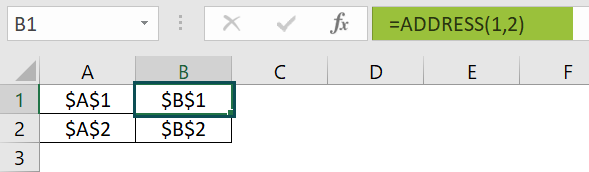Select cell A2, enter the formula =ADDRESS(2,1), and the result is “\$A\$2”.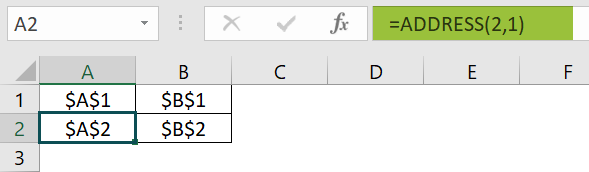In cell B2, enter the formula =ADDRESS(2,2), and the result is “\$B\$2”.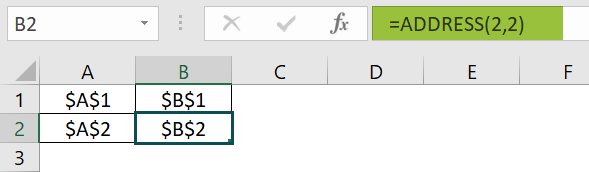The cell addresses are shown in the images.

2. What is the usage of the ADDRESS function in Excel?

The ADDRESS Function in Excel is used to,
Find the cell reference value from the row and column numbers.
Convert a column number to a letter and vice versa.
Builds a cell reference within the ADDRESS formula.
Return the address of the cell with the highest value.
Find the address of the first or the last cell in a range.

3. Where is the ADDRESS function in Excel?

First, choose an empty cell for the output → select the “Formulas” tab → go to the “Function Library” group → click the “Lookup & Reference” drop-down → select the “ADDRESS” function as shown below.